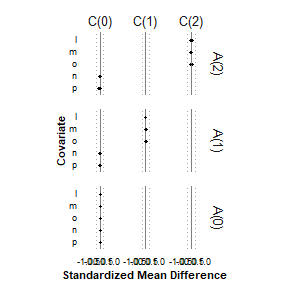# Overview

Below, we provide an example in which we apply Diagnostic 3 to an artificial data set to illustrate covariate balance for a time-varying exposure without censoring.

# Load example data and packages

data("example_sml")

## Example: Diagnostic 3 for a time-varying exposure without censoring

### PRELIMINARY STEP: MAKE EXPOSURE HISTORY

##### Remove history for illustration. Note that the input data is already in wide format.
drops <- c("h_0", "h_1", "h_2")
mydata <- example_sml[ , !(names(example_sml) %in% drops)]

mydata.history <- makehistory.one(input=mydata,
id="id",
exposure="a",
name.history="h",
times=c(0,1,2))

### STEP 1: RESTRUCTURE THE DATA

mydata.tidy <- lengthen(
input=example_sml, #mydata.history,
id="id",
diagnostic=3,
censoring="no",
times.exposure=c(0,1,2),
times.covariate=c(0,1,2),
exposure="a",
temporal.covariate=c("l","m","o"),
static.covariate=c("n","p"),
history="h",
weight.exposure="wax"
)

### Example of how to remove relative covariate history

mydata.tidy.omit <- omit.history(
input=mydata.tidy,
omission="relative",
covariate.name=c("l","m","o"),
distance=1
)

### STEP 2: CREATE BALANCE TABLE

mytable <- balance (
input=mydata.tidy.omit,
diagnostic=3,
approach="weight",
censoring="no",
scope="all",
times.exposure=c(0,1,2),
times.covariate=c(0,1,2),
exposure="a",
history="h",
weight.exposure="wax",
ignore.missing.metric="no",
sort.order= c("l","m","o","n","p")
)

### STEP 3: PLOT BALANCE METRIC

myplot <- makeplot (
input=mytable,
diagnostic  =3,
approach="weight",
scope="all",
metric="SMD"
)

### Display the plot

myplot### STEP 4: SAVE BALANCE TABLE AND PLOT

write.csv(mytable,paste(path,"mytable.csv",sep=""))
ggsave(filename=paste(path,"myplot.pdf",sep=""))

## Example of Regression Approach for Diagnostic 1

Now, we illustrate how Diagnostic 1 can be implemented using a regression approach.

### Create tidy dataset

mydata.tidy <- lengthen(
input=mydata.history,
diagnostic=1,
censoring="no",
id="id",
times.exposure=c(0,1,2),
times.covariate=c(0,1,2),
exposure="a",
temporal.covariate=c("l","m","n","o","p"),
history="h"
)

head(mydata.tidy)
#>   id name.cov time.exposure time.covariate   h a value.cov
#> 1  1        l             0              0   H 1         1
#> 2  1        l             1              0  H1 0         1
#> 3  1        l             1              1  H1 0         1
#> 4  1        l             2              0 H10 0         1
#> 5  1        l             2              1 H10 0         1
#> 6  1        l             2              2 H10 0         1

Notice that lengthen has already subset the data appropriately for diagnostic 1, where at any time $$t$$ covariates are measured at or before exposure. We could use omit.history() to further restrict to the same measurement times for exposures and covariates.

### Make a balance table using lengthen() and regression models


library(dplyr)
#>
#> Attaching package: 'dplyr'
#> The following objects are masked from 'package:stats':
#>
#>     filter, lag
#> The following objects are masked from 'package:base':
#>
#>     intersect, setdiff, setequal, union
library(broom)

mydata.tidy.reg <- mutate(mydata.tidy,
time=time.exposure,
distance=time.exposure-time.covariate,
history=h)

output <- mydata.tidy.reg %>%
group_by(name.cov) %>% #note, you can include other stratifying variables here or in the model
filter(time.exposure>=time.covariate) %>% #lengthen actually arealdy took care of this, provided here for clarity
do(tidy(lm(formula=value.cov~a+time+distance+history,.))) %>% #same model form used for every covariate
filter(term=="a") %>% ungroup()

table.reg <- output %>%
select(name.cov,estimate) %>%
rename(D=estimate)

print(table.reg)
#> # A tibble: 5 x 2
#>   name.cov       D
#>   <chr>      <dbl>
#> 1 l         0.102
#> 2 m        -0.149
#> 3 n         0.0462
#> 4 o         0.0832
#> 5 p         0.0368

### Compare that to a balance table via direct calculation & standardization


table.std <- balance(input=mydata.tidy,
diagnostic=1,
approach="none",
censoring="no",
scope="average",
average.over="distance",
ignore.missing.metric="no",
times.exposure=c(0,1,2),
times.covariate=c(0,1,2),
exposure="a",
history="h"
)

print(table.std)
#>   period.id period.start period.end name.cov           D         SMD     N
#> 1         1            0          2        l  0.10203234  0.21905115 60000
#> 2         1            0          2        m -0.15051552 -0.33489936 60000
#> 3         1            0          2        n  0.04648841  0.09452689 60000
#> 4         1            0          2        o  0.08324444  0.18305066 60000
#> 5         1            0          2        p  0.03766586  0.08452254 60000